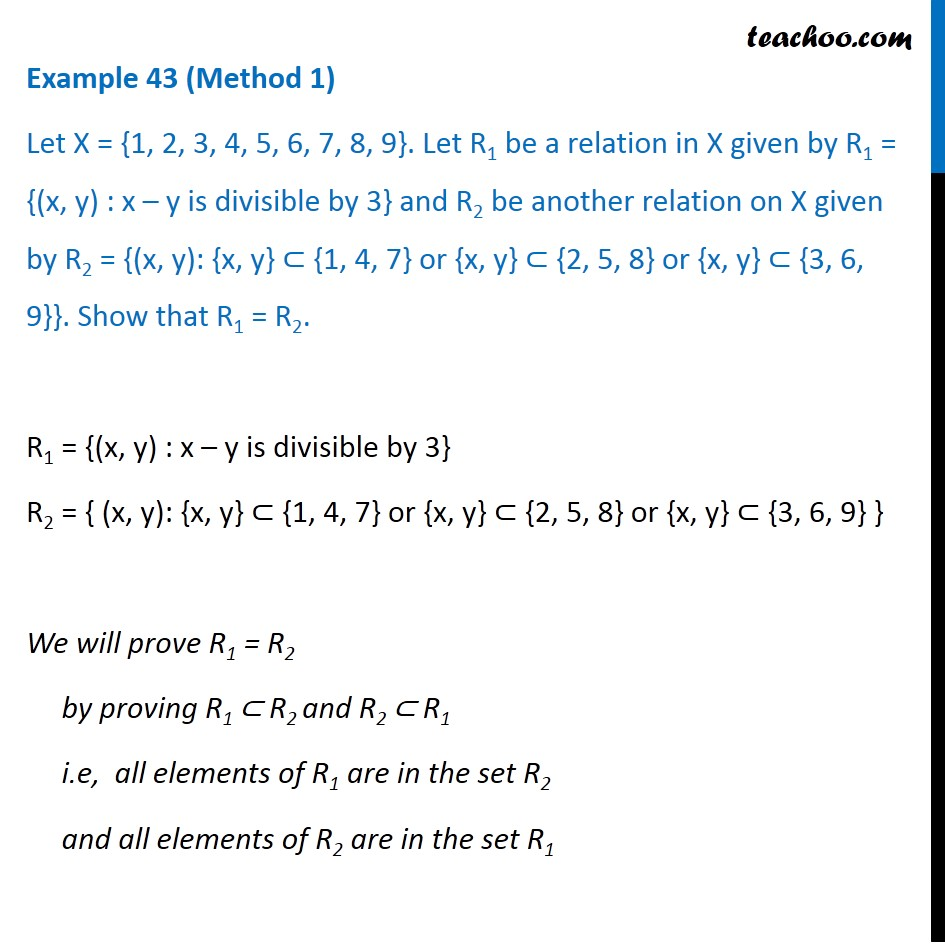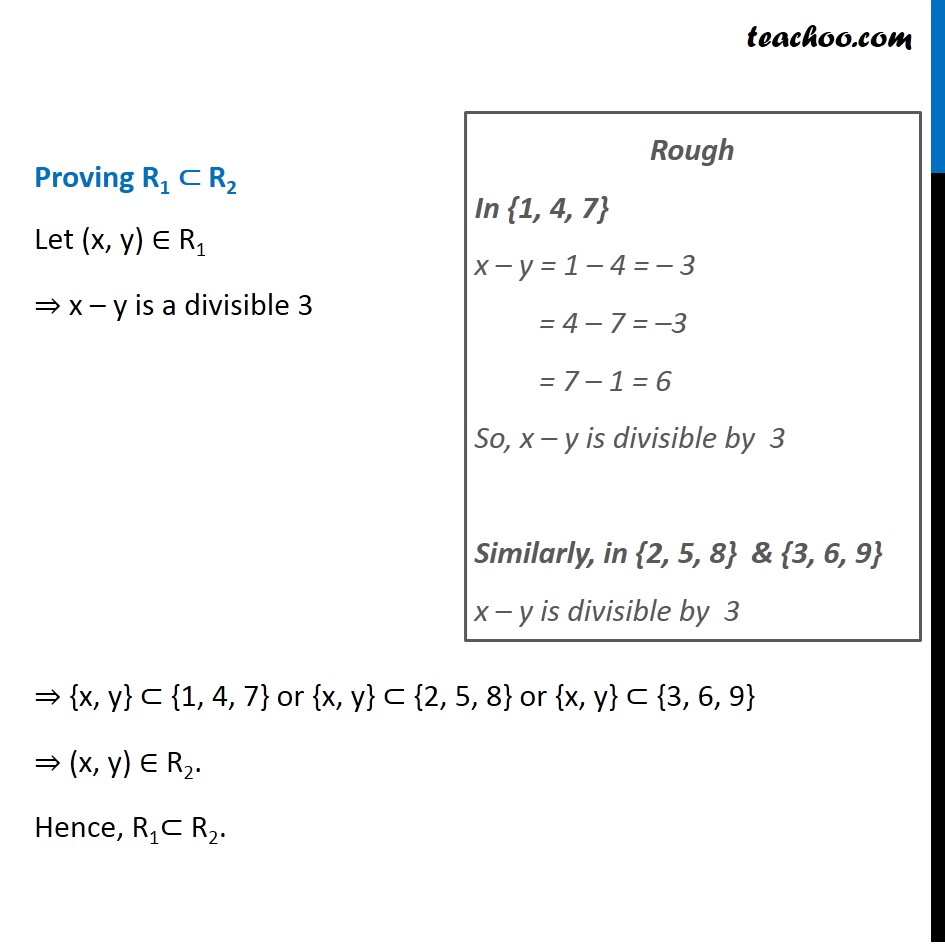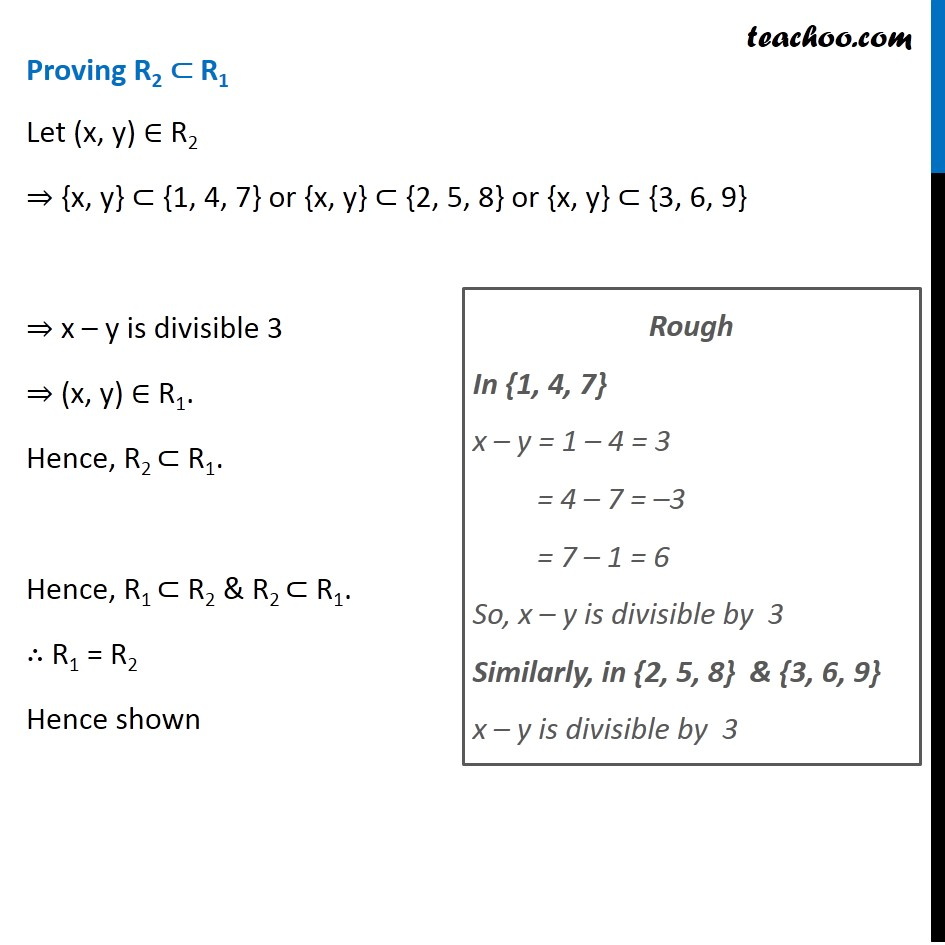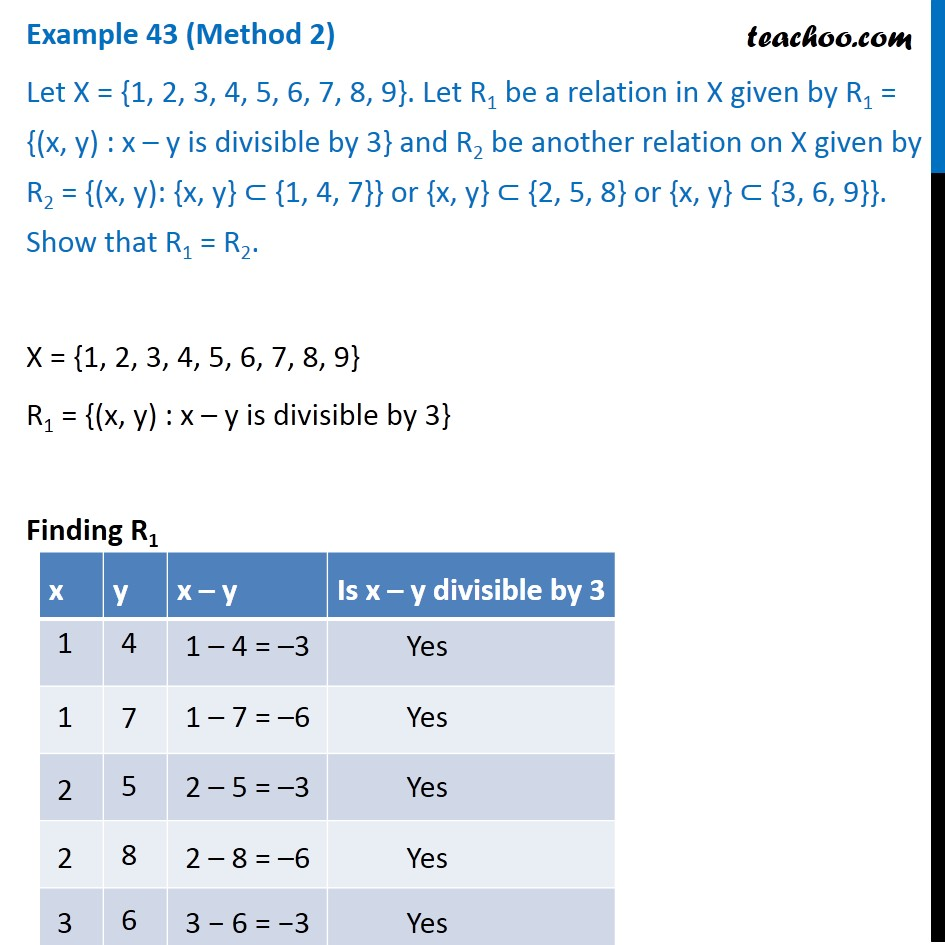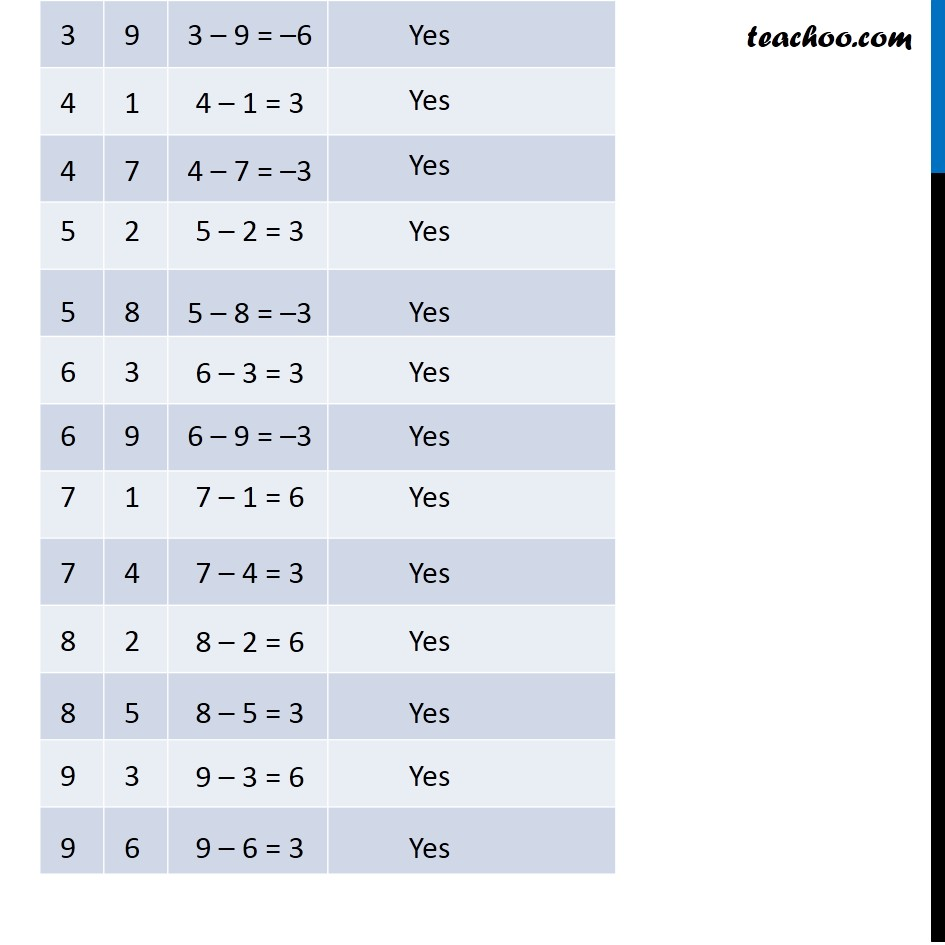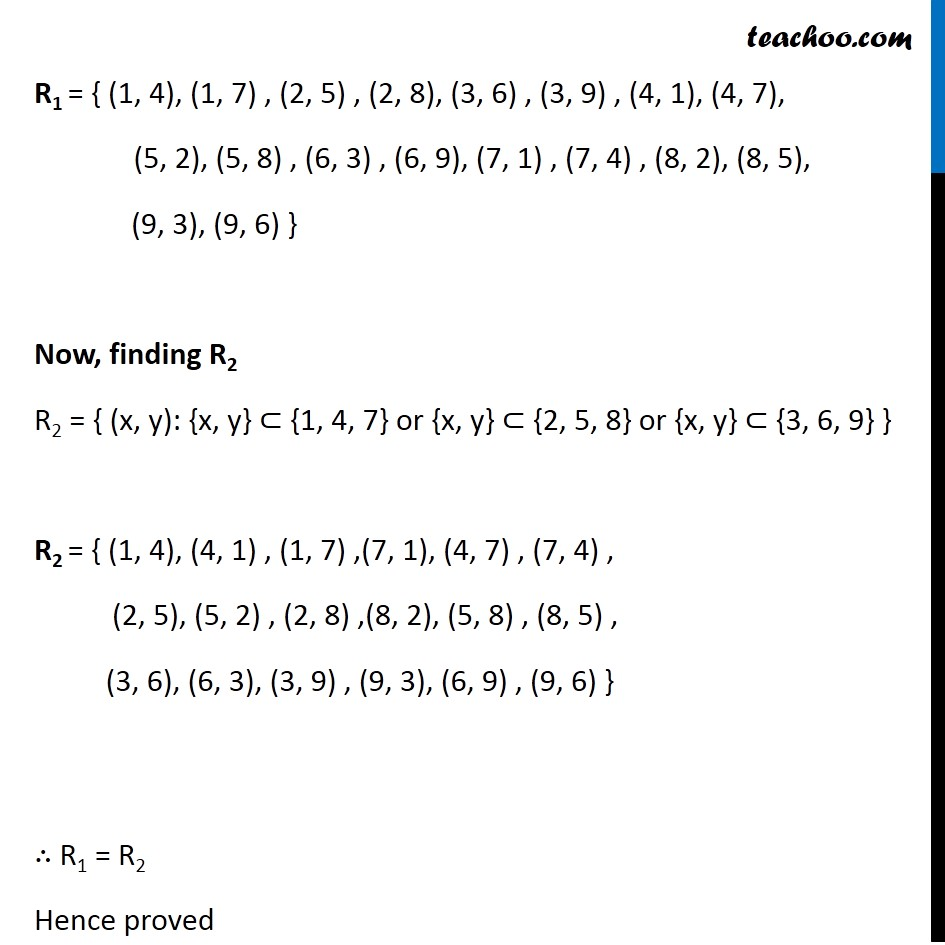Subscribe to our Youtube Channel - https://you.tube/teachoo

1. Chapter 1 Class 12 Relation and Functions
2. Concept wise
3. Relations - Definition

Transcript

Example 43 (Method 1) Let X = {1, 2, 3, 4, 5, 6, 7, 8, 9}. Let R1 be a relation in X given by R1 = {(x, y) : x – y is divisible by 3} and R2 be another relation on X given by R2 = {(x, y): {x, y} ⊂ {1, 4, 7} or {x, y} ⊂ {2, 5, 8} or {x, y} ⊂ {3, 6, 9}}. Show that R1 = R2. R1 = {(x, y) : x – y is divisible by 3} R2 = { (x, y): {x, y} ⊂ {1, 4, 7} or {x, y} ⊂ {2, 5, 8} or {x, y} ⊂ {3, 6, 9} } We will prove R1 = R2 by proving R1 ⊂ R2 and R2 ⊂ R1 i.e, all elements of R1 are in the set R2 and all elements of R2 are in the set R1 Proving R1 ⊂ R2 Let (x, y) ∈ R1 ⇒ x – y is a divisible 3 ⇒ {x, y} ⊂ {1, 4, 7} or {x, y} ⊂ {2, 5, 8} or {x, y} ⊂ {3, 6, 9} ⇒ (x, y) ∈ R2. Hence, R1⊂ R2. Rough In {1, 4, 7} x – y = 1 – 4 = – 3 = 4 – 7 = –3 = 7 – 1 = 6 So, x – y is divisible by 3 Similarly, in {2, 5, 8} & {3, 6, 9} x – y is divisible by 3 Proving R2 ⊂ R1 Let (x, y) ∈ R2 ⇒ {x, y} ⊂ {1, 4, 7} or {x, y} ⊂ {2, 5, 8} or {x, y} ⊂ {3, 6, 9} ⇒ x – y is divisible 3 ⇒ (x, y) ∈ R1. Hence, R2 ⊂ R1. Hence, R1 ⊂ R2 & R2 ⊂ R1. ∴ R1 = R2 Hence shown Rough In {1, 4, 7} x – y = 1 – 4 = 3 = 4 – 7 = –3 = 7 – 1 = 6 So, x – y is divisible by 3 Similarly, in {2, 5, 8} & {3, 6, 9} x – y is divisible by 3 Example 43 (Method 2) Let X = {1, 2, 3, 4, 5, 6, 7, 8, 9}. Let R1 be a relation in X given by R1 = {(x, y) : x – y is divisible by 3} and R2 be another relation on X given by R2 = {(x, y): {x, y} ⊂ {1, 4, 7}} or {x, y} ⊂ {2, 5, 8} or {x, y} ⊂ {3, 6, 9}}. Show that R1 = R2. X = {1, 2, 3, 4, 5, 6, 7, 8, 9} R1 = {(x, y) : x – y is divisible by 3} Finding R1 R1 = { (1, 4), (1, 7) , (2, 5) , (2, 8), (3, 6) , (3, 9) , (4, 1), (4, 7), (5, 2), (5, 8) , (6, 3) , (6, 9), (7, 1) , (7, 4) , (8, 2), (8, 5), (9, 3), (9, 6) } Now, finding R2 R2 = { (x, y): {x, y} ⊂ {1, 4, 7} or {x, y} ⊂ {2, 5, 8} or {x, y} ⊂ {3, 6, 9} } R2 = { (1, 4), (4, 1) , (1, 7) ,(7, 1), (4, 7) , (7, 4) , (2, 5), (5, 2) , (2, 8) ,(8, 2), (5, 8) , (8, 5) , (3, 6), (6, 3), (3, 9) , (9, 3), (6, 9) , (9, 6) } ∴ R1 = R2 Hence proved

Relations - Definition